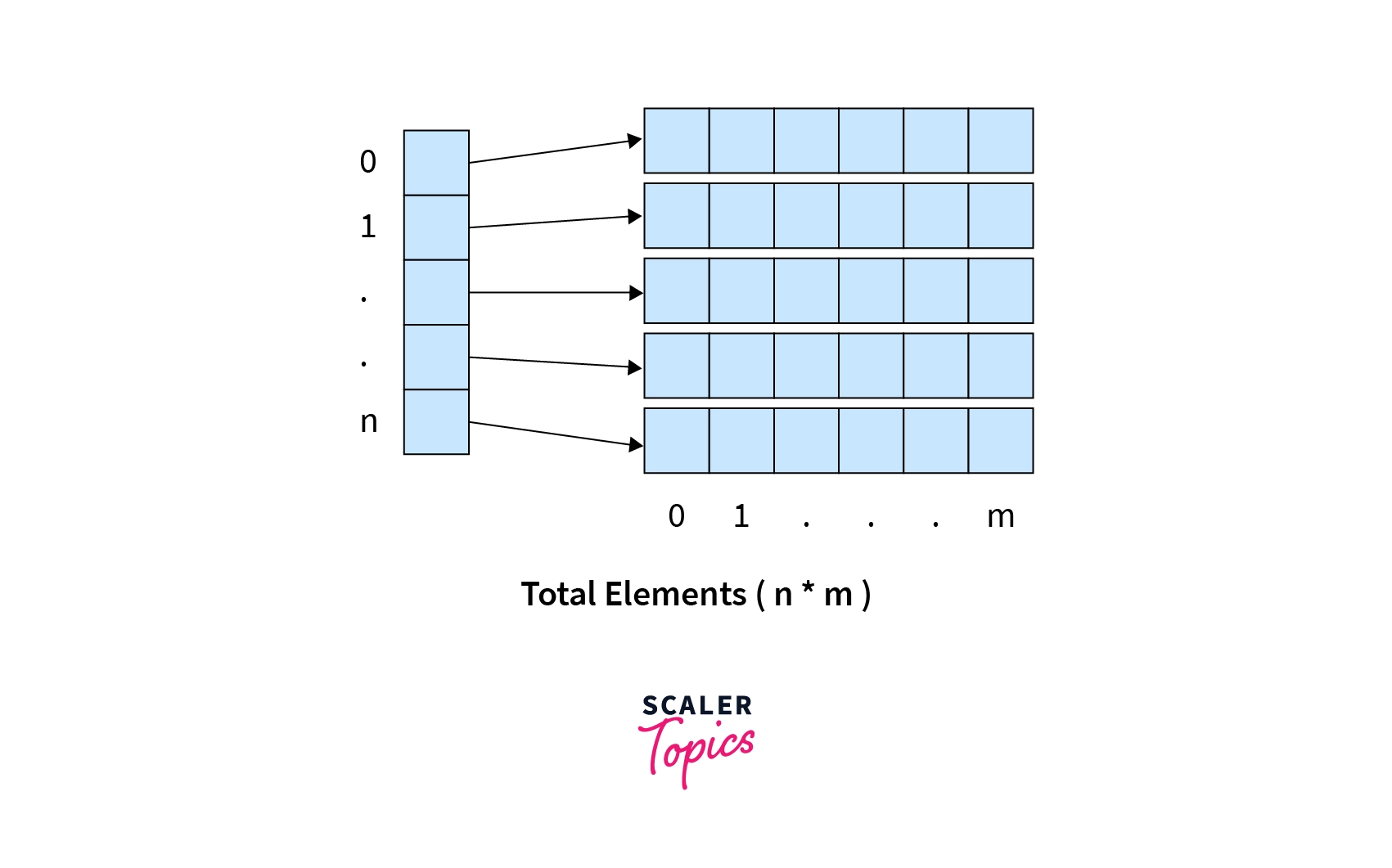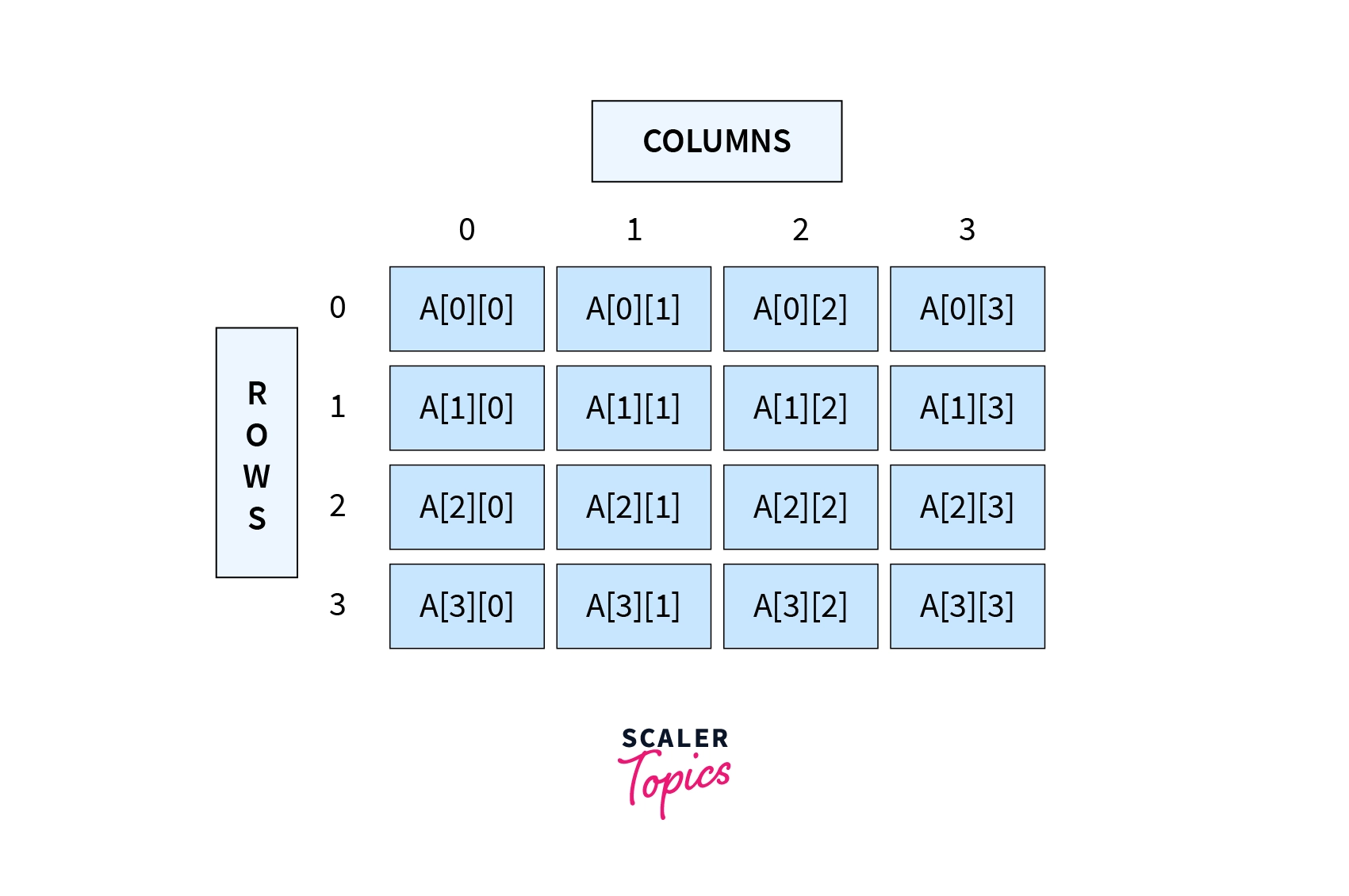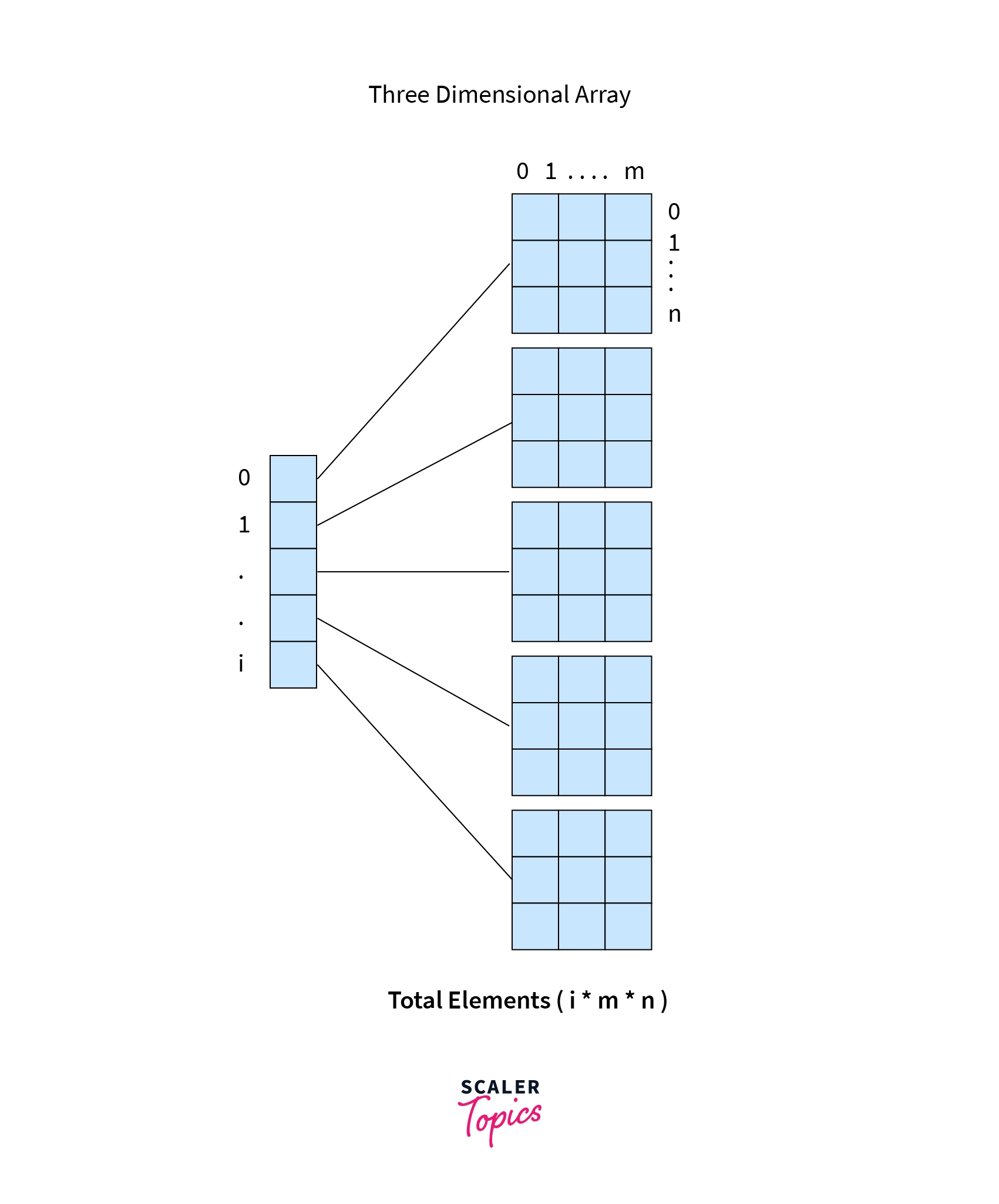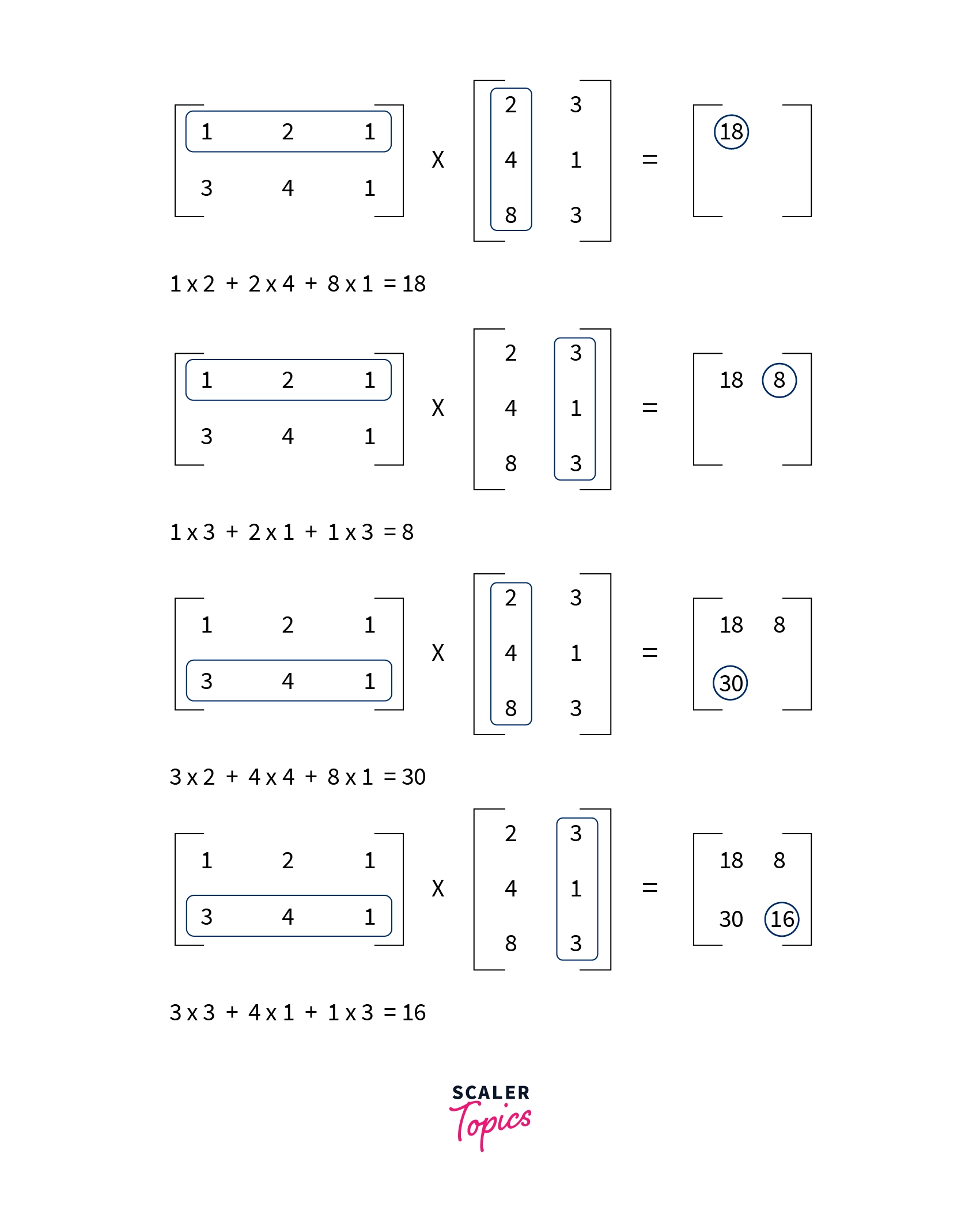# Multidimensional Arrays in Java

Challenge Inside! : Find out where you stand! Try quiz, solve problems & win rewards!
Learn via video courseJava Course - Mastering the Fundamentals
By Tarun LuthraFree
5Enrolled: 1000Java Course - Mastering the Fundamentals
Tarun LuthraFree
5
Enrolled: 1000

## Overview

An array is a homogeneous data structure that stores data of the same type in contiguous memory locations. Multidimensional Arrays can be thought of as an array inside the array i.e. elements inside a multidimensional array are arrays themselves. They can hold more than one row and column in tabular form. Java supports multidimensional arrays that are two, three, four, five, or more levels deep. However, arrays more than three levels deep are hard to manage.

## Introduction to Multidimensional Array in Java

An array is a data structure to store a collection of values of the same type. The data can be accessed using its integer index. Since all the data is stored in contiguous memory locations, an array is always initialized with its size, and it cannot be changed while the program is running.

Multidimensional arrays, like a 2D array is a bunch of 1D arrays put together in an array. A 3D array is like a bunch of 2D arrays put together in a 1D array, and so on. Data in multi-dimensional arrays are stored in row-major format, i.e., tabular form. To access array elements in multidimensional arrays, more than one index is used.

Note: Java doesn't have multi-dimensional arrays. They are nested arrays.## Syntax of Multidimensional Array in Java

The general syntax to declare a multi-dimensional array

General syntax to initialize the array:

Or

Here, DataType is the type of data to be stored in the array. The array can be 1 dimensional to N-dimensional. arrayName is the variable name given to the array and length is the size of the array of respective dimensions.

## Types of Multidimensional Array in Java

Multidimensional array can be a 2D array, a 3D array, a 4D array, where D stands for Dimension. But the higher the dimension, the more difficult it is to access and store elements.

We will discuss 2D Array in Java and 3D Array in Java in detail.

### 1. Two-Dimensional Array or 2D Array.

Many computer games, like simulation games, strategy games, or first-person conflict games, involve objects that occupy a two-dimensional space. Applications for such positional games use a two-dimensional array for representing objects using indices, say i and j, that refer to the cells in the array. The first index refers to a row number, and the second index refers to a column number. In Java, a two-dimensional array is nothing but a table or matrix with columns and rows. It is the simplest multi-dimensional array.

a. Indirect Method of Declaration

The indirect method is the method where the size of the array is declared, and array values are filled later. The two square brackets ([][]) can be written either after DataType or after arrayName.

Syntax to initialize and declare a multidimensional array.

A two-dimensional array can be declared as follows:

Initialization:

Java is a statically-typed programming language, specifying DataType decides the type of element it will accept. arrayName is the variable name given to the array which will be used while accessing, manipulating, and storing data. length 1 is the number of rows in a two-dimensional array, and length 2 is the number of columns in it.

Here, initially, all the array cells are filled with some base or garbage values.

Note: Size of a two-dimensional array is $length 1 * length 2$, i.e. number of elements in an array is $length 1 * length 2$.

Example:

Output:

Note that indexing in java starts with 0.

Here, we first declare and initialize a two-dimensional array named twoD_array of int dataType. Its total rows are 3, and total columns are 4 as in initialization int. The Row number is always written first and then the column number. In the first for loop, row is the row number, and in the inner loop, col is the column number.

twoD_array.length returns the number of rows in our array. And twoD_array[row].length gives the length of the particular row in the array. Generally, for the number of columns in an array, twoD_array, that is, 0th index is used. Then we print the array using enhanced for loop. The outer loop takes each int[] array as a temp from twoD_array, and the inner loop takes each element from that temp array and prints it.

b. Direct Method of Declaration

The direct method is where values to the array are assigned during declaration only.

Syntax to initialize and declare a multidimensional array:

Note: The two square brackets([][]) can be written either after DataType or after arrayName while declaring the two-dimensional array.

Example:

Output:

In the above example, we initialize the element values directly. Here, we don't have to write the size of the array.

There are various approaches to initializing the array:

• In the first example, we initialized the array using for loops.
• In the second example, we directly initialized the values only. Here compiler automatically calculates the rows and columns of the array.
• We can also assign values at various positions separately. This method is difficult to use when there is a large array.

For Example:

Output:

Jagged Arrays

In all the examples we have seen so far, we had fixed number of columns, and each subarray or row of the two-dimensional array had the same number of elements. Java provides a concept of Jagged Arrays where a two-dimensional array can have sub-arrays of different sizes.

Output:

It is a two-dimensional array only, so all the methods and initializations are the same. It can have empty arrays as well.

c. Accessing Elements of Two-Dimensional Arrays

Elements of a Two-dimensional array can be accessed using row numbers and column numbers. For example,

For example:

This will return the element at the 0th row and 2nd column.

Printing elements of two-dimensional Arrays

• Simplest way to print elements is directly accessing elements
• It can also be accessed using for loop:
• Enhanced for loop or for-each loop can also be used to access elements

d. Representation of 2D arraye. Example: Addition of two matrices

To add two arrays, we create 2 arrays, matrixA and matrixB and initialize them. A third array, matrixC is the array that we obtain by the addition of matrixA and matrixB.

Note: For addition, all the three matrices should have the same number of rows and columns.

Output:

### 2. Three-Dimensional Array or 3D Array.

An array is a complex form of a multidimensional array. A three-dimensional array adds an extra dimension and exponentially increases the size of a two-dimensional array. Effectively it is an array containing multiple two-dimensional arrays, with each element addressed by three indices, the first one for a two-dimensional array` and the rest two for the rows and the columns.

a. Indirect Method of Declaration

Syntax to declare a three-dimensional array:

Initialization:

It can be of int, String, char, float, etc. DataTypes. Number of elements in a three-dimensional array is "length 1 * length 2 * length 3". And memory usage is equal to "number of elements * size of datatype". For instance, the size of int datatype in Java is 4 bytes. Thus, memory usage is 4 * (number of elements in an array) bytes.

In this type of initialization, java fills all the cells of the array with some base or garbage values. For int array, it fills them with zero(0).

Example:

Number of elements in this array is 8.

b. Direct Method of Declaration

c. Accessing Elements of Three-Dimensional Arrays

Elements in three-dimensional arrays can be accessed as

This is similar to accessing a two-dimensional array. Here, arrayIndex is the outer array index, and rowIndex and columnIndex are rows and columns of inner arrays, respectively.

Printing elements of three-dimensional Arrays Three-Dimensional arrays can also be printed using for loops, for-each loops, and individually.

• Simplest way to print elements is directly accessing elements
• It can also be accessed using for loop:
• Enhanced for loop or for-each loop can also be used to access elements

d. Representation of 3D arraye. Example of Three-Dimensional Array In the given example, we have data of 3 students, and their scores of 4 subjects in two semesters. As we can see, all three parameters are interrelated and can be stored in a single three-dimensional array. The outermost array can store the details student-wise. The inner array is nothing but a 2D array of semesters and subject scores. Each student has a unique semester and subject table. This unique table is then stored in our three-dimensional array.

Similarly, the data can be accessed using for loops. The outermost loop will access the student number, the first inner loop will access the semester number, and the innermost loop will print the scores subject-wise.

Output:

## Operations on Multidimensional Arrays

Many operations can be performed on a multidimensional array. We can add them, subtract them, multiply them. We already saw the addition of arrays in the two-dimensional array. Here in this section, we will see the multiplication of two-dimensional arrays similar to matrix multiplication.Output:

Firstly we take two matrices, and we check if the number of rows of the 1st array is equal to the number of columns of the 2nd array. If it is not true, multiplication cannot be performed, and hence we return that it is not possible. Next, we do the usual multiplication of the arrays using for loop. And at last, we print the array.

## Conclusion

• Arrays are homogenous data structures that store the same type of data in them.
• Arrays can be single dimensional or multidimensional.
• Multidimensional arrays are a little complicated, and complexity increases with an increase in dimensions.
• Multidimensional arrays can be two-dimensional, three-dimensional, etc.
• Two-dimensional array can be visualized as an array of 1D arrays. Likewise, a Three-dimensional array can be considered an array of 2D arrays.
• Elements can be added to the array in two ways, directly and indirectly.
• They can be accessed in multiple ways, like using loops or using indexes.
• Two-dimension and Three-dimension arrays have many applications in graphics, games, software, mathematical analysis, etc.

## FAQs

Q. What is a Single-dimensional array in Java?

A. A Single-dimensional array is a sequenced collection of variables all of the same type. In other words, it is a linear data structure to store similar types of elements having contiguous index-based memory locations. Each value stored in an array is called an element, and each element in an array has an index, which uniquely refers to the value stored. A one-dimensional array is used to store a fixed set of elements.

Q. What is a two-dimensional array?

A. Two-dimensional arrays in Java are like creating an array of arrays. A two-dimensional array is an array with each of its cells filled with another array. It is also referred to as a matrix. 2D arrays have many applications in numerical analysis, computer games, etc.

Q. What does it mean to be two-dimensional?

A. Being two-dimensional means having two dimensions like a rectangle shape has two dimensions i.e. length and breadth. A two-dimensional array can be considered an array that stores arrays of the same data type. It is also called “an array of arrays". Two-dimensional arrays can be represented as a collection of rows and columns.

Q. Which one comes first in an array – Rows or Columns?

A. Usually, the horizontal axis (X) comes before the vertical axis (Y). However, in java or programming languages, it is the opposite. While substituting value in a two-dimensional array or retrieving the value from it, row is written first and then the column. For instance, A where 8 refers to the row number and 9 refers to the column number. Although while declaring a two-dimensional array, mentioning the number of rows is a must but mentioning number of columns is optional.

Q. Why would you need a multidimensional array in Java?

A. Multi-dimensional arrays have many applications in mathematics and numerical analysis. One might question that when we have a one-dimensional array, then why do we need multi-dimensional arrays? We have both a Character array and Strings in java. Both perform the same purpose, but it is easy and convenient to use String as compared to a Character array. Similarly, we have variables, but we use data structures to store thousands of values. It also reduces code complexity.

Q. When would you use a multidimensional array?

A. Imagine you have to save your scores of mid-term and end-term of 5 subjects. Create an array with 2 rows and 5 columns where rows denote term and columns denote subject. It is used while dealing with graphics and pixels. It could also act as a spreadsheet.